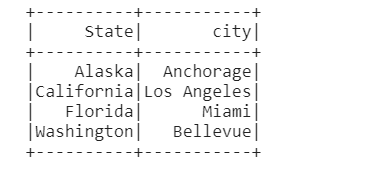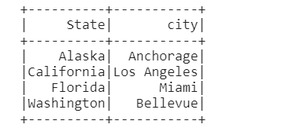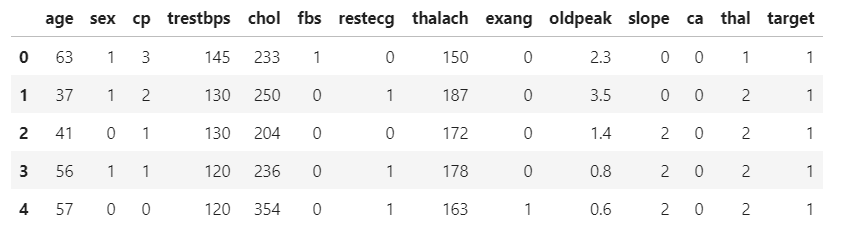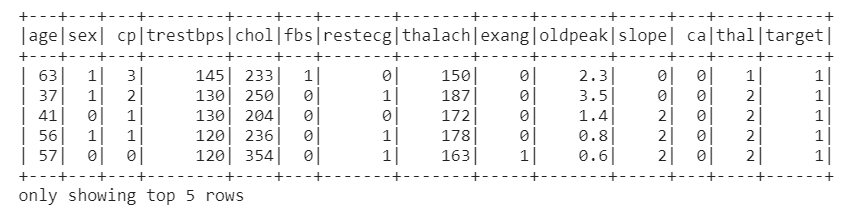Related Articles

# How to Convert Pandas to PySpark DataFrame ?

• Last Updated : 23 May, 2021

In this article, we will learn How to Convert Pandas to PySpark DataFrame. Sometimes we will get csv, xlsx, etc. format data, and we have to store it in PySpark DataFrame and that can be done by loading data in Pandas then converted PySpark DataFrame. For conversion, we pass the Pandas dataframe into the CreateDataFrame() method.

Syntax: spark.createDataframe(data, schema)

Attention geek! Strengthen your foundations with the Python Programming Foundation Course and learn the basics.

To begin with, your interview preparations Enhance your Data Structures concepts with the Python DS Course. And to begin with your Machine Learning Journey, join the Machine Learning - Basic Level Course

Parameter:

• data – list of values on which dataframe is created.
• schema – It’s the structure of dataset or list of column names.

where spark is the SparkSession object.

Example 1: Create a DataFrame and then Convert using spark.createDataFrame() method

## Python3

 `# import the pandas``import` `pandas as pd`` ` `# from  pyspark library import ``# SparkSession``from` `pyspark.sql ``import` `SparkSession`` ` `# Building the SparkSession and name``# it :'pandas to spark'``spark ``=` `SparkSession.builder.appName(``  ``"pandas to spark"``).getOrCreate()`` ` `# Create the DataFrame with the help ``# of pd.DataFrame()``data ``=` `pd.DataFrame({``'State'``: [``'Alaska'``, ``'California'``,``                               ``'Florida'``, ``'Washington'``],``                      ` `                     ``'city'``: [``"Anchorage"``, ``"Los Angeles"``, ``                              ``"Miami"``, ``"Bellevue"``]})`` ` `# create DataFrame``df_spark ``=` `spark.createDataFrame(data)`` ` `df_spark.show()`

Output:Example 2: Create a DataFrame and then Convert using spark.createDataFrame() method

In this method, we are using Apache Arrow to convert Pandas to Pyspark DataFrame.

## Python3

 `import` `the pandas``import` `pandas as pd`` ` `# from  pyspark library import ``# SparkSession``from` `pyspark.sql ``import` `SparkSession`` ` `# Building the SparkSession and name ``# it :'pandas to spark'``spark ``=` `SparkSession.builder.appName(``  ``"pandas to spark"``).getOrCreate()`` ` `# Create the DataFrame with the help ``# of pd.DataFrame()``data ``=` `pd.DataFrame({``'State'``: [``'Alaska'``, ``'California'``,``                               ``'Florida'``, ``'Washington'``],``                      ` `                     ``'city'``: [``"Anchorage"``, ``"Los Angeles"``,``                              ``"Miami"``, ``"Bellevue"``]})`` ` ` ` `# enableing the Apache Arrow for converting``# Pandas to pySpark DF(DataFrame)``spark.conf.``set``(``"spark.sql.execution.arrow.enabled"``, ``"true"``)`` ` `# Creating the DataFrame``sprak_arrow ``=` `spark.createDataFrame(data)`` ` `# Show the DataFrame``sprak_arrow.show()`

Output:Example 3: Load a DataFrame from CSV and then Convert

In this method, we can easily read the CSV file in Pandas Dataframe as well as in Pyspark Dataframe. The dataset used here is heart.csv.

## Python3

 `# import the pandas library``import` `pandas as pd`` ` `# Read the Dataset in Pandas Dataframe``df_pd ``=` `pd.read_csv(``'heart.csv'``)`` ` `# Show the dataset here head() ``# will return top 5 rows``df_pd.head()`

Output:## Python3

 `# Reading the csv file in ``# Pyspark DataFrame``df_spark2 ``=` `spark.read.option(``  ``'header'``, ``'true'``).csv(``"heart.csv"``)`` ` `# Showing the data in the from of ``# table and showing only top 5 rows``df_spark2.show(``5``)`

Output:We can also convert pyspark Dataframe to pandas Dataframe. For this, we will use DataFrame.toPandas() method.

Syntax: DataFrame.toPandas()

Returns the contents of this DataFrame as Pandas pandas.DataFrame.

## Python3

 `# Convert Pyspark DataFrame to ``# Pandas DataFrame by toPandas() ``# Function head() will show only``# top 5 rows of the dataset``df_spark2.toPandas().head()`

Output:My Personal Notes arrow_drop_up